## RD Sharma Class 10 Solutions Chapter 4 Quadratic Equations Ex 4.5

These Solutions are part of RD Sharma Class 10 Solutions. Here we have given RD Sharma Class 10 Solutions Chapter 4 Quadratic Equations Ex 4.5

Other Exercises

Question 1.
Write the discriminant of the following quadratic equations :
(i) 2x² – 5x + 3 = 0
(ii) x² + 2x + 4 = 0
(iii) (x – 1) (2x – 1) = 0
(iv) x² – 2x + k = 0, k ∈ R
(v) √3 x² + 2√2 x – 2√3 = 0
(vi) x² – x + 1 = 0
Solution: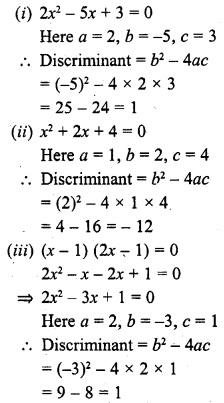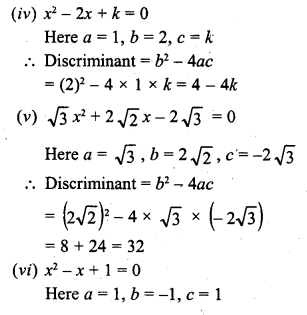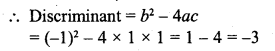Question 2.
In the following, determine whether the given quadratic equations have real roots and if so, And the roots :
(i) 16x² = 24x + 1
(ii) x² + x + 2 = 0
(iii) √3 x² + 10x – 8√3 = 0
(iv) 3x² – 2x + 2 = 0
(v) 2x² – 2√6 x + 3 = 0
(vi) 3a²x² + 8abx + 4b² = 0, a ≠ 0
(vii) 3x² + 2√5 x – 5 = 0
(viii) x² – 2x + 1 = 0
(ix) 2x² + 5√3 x + 6 = 0
(x) √2 x² + 7x + 5√2= 0 [NCERT]
(xi) 2x² – 2√2 x + 1 = 0 [NCERT]
(xii) 3x² – 5x + 2 = 0 [NCERT]
Solution: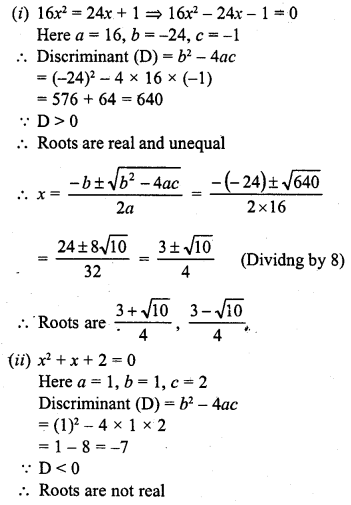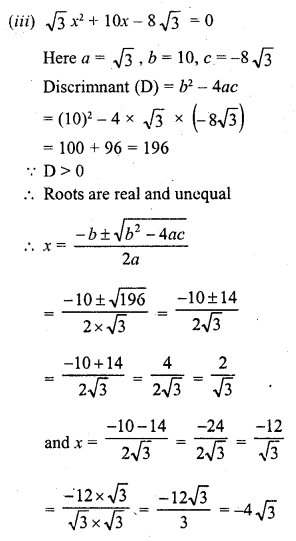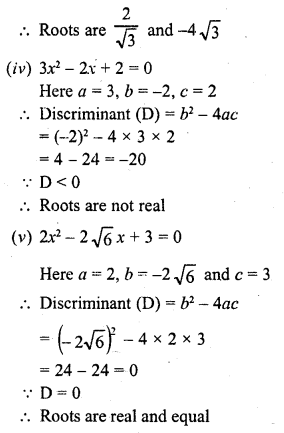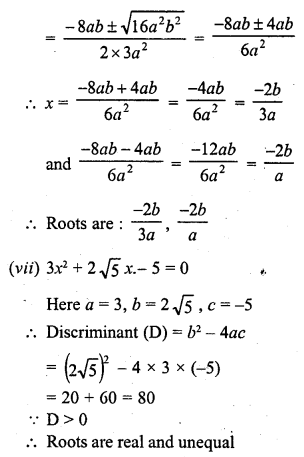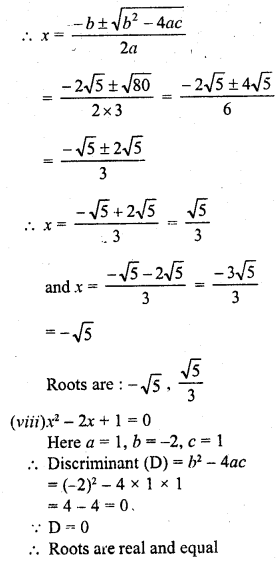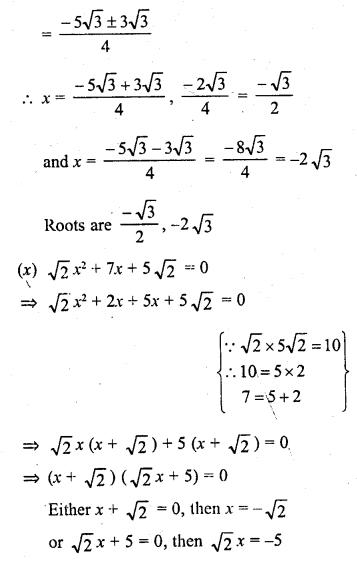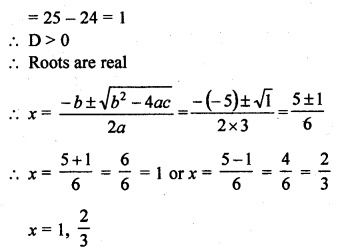Question 3.
Solve for x :Solution: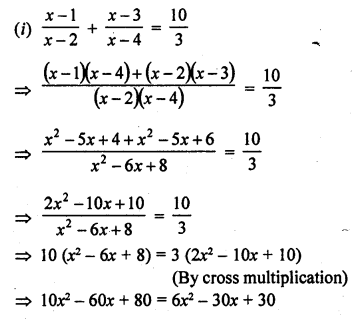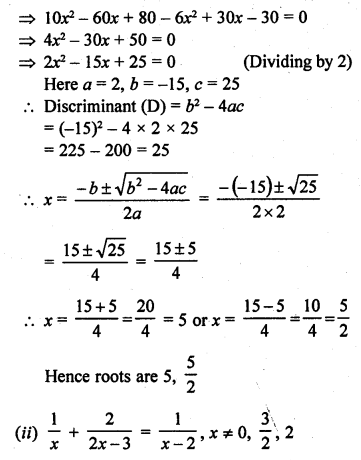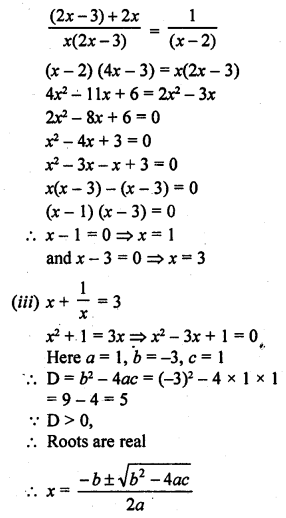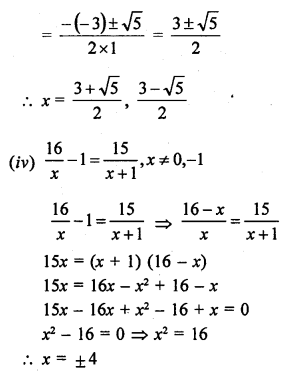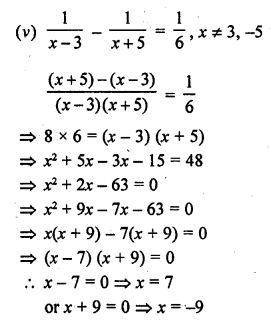Hope given RD Sharma Class 10 Solutions Chapter 4 Quadratic Equations Ex 4.5 are helpful to complete your math homework.

If you have any doubts, please comment below. Learn Insta try to provide online math tutoring for you.